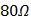# In the adjoining circuit, the e.m.f.of the cell isand the internal resistance is negligible. The resistance of the voltmeter isThe reading of the voltmeter will bea)b)c)d)## Question ID - 150367 :- In the adjoining circuit, the e.m.f.of the cell isand the internal resistance is negligible. The resistance of the voltmeter isThe reading of the voltmeter will bea)b)c)d)3537

 (c) Total resistance of the circuitMain currentCombination of voltmeter andresistance is connected in series with, so current throughand this combination will be sameSince the resistance of voltmeter is also, so this current is equally distributed inresistance and voltmeter [through each] P.D. acrossresistance5 cells, each of emfand internal resistanceare connected to an external circuit of resistance ofFind the current through external circuit a)b)c)d)